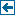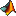Master index Index for chronux/spectral_analysis/plots# Index for chronux/spectral_analysis/plots

## Matlab files in this directory:plot_matrix Function to plot a time-frequency matrix X. Time and frequency axes are in t and f.plot_vector Function to plot a frequency dependent vector X. If error bars are specified in Xerr,plotsig Function to plot C where it is higher than a threshold sigplotsigdiff Function to plot significant differences between two time-frequency arrays X1 and X2

Generated on Fri 28-Sep-2012 12:34:27 by m2html © 2005